# Catch up on the latest AI articles# GaCNN : Optimize CNN Architecture With Genetic Algorithm (GA)!

3 main points
✔️ Develop a computationally efficient GA to evolve CNN
✔️ Validated on FashionMNIST and MNIST, outperforming the accuracy of 12 out of 16 competing methods
✔️ Successfully compressed optimization that used to take more than a few days into less than a day

(Submitted on 28 Jul 2021)
Comments:Published in 2021 IEEE Congress on Evolutionary Computation (CEC)
Subjects: Computer Vision and Pattern Recognition (cs.CV)

The images used in this article are from the paper, the introductory slides, or were created based on them.

## background

The CNN architecture has been improved remarkably in recent years, and the classification accuracy has increased along with it! However, while the architecture of CNNs is becoming more complex, the optimization of the architecture is increasingly dependent on "human power".

So, I'm going to introduce a paper that proposes "gaCNN" as a method to optimize CNN architecture with GA (Genetic Algorithm)!

The main elements described in the proposed methodology are

• gene encoding strategy
• Mutation operator
• Heterogeneous activation functions
• Fitness evaluation Strategies

+ Efficiently identify good CNNs architectures tested on benchmark datasets.

## related research

To evolve a CNN using GAs, we need to get the genetic math right!

### Proposed encoding strategy for two genes representing CNNs (Sun et al.)

1st gene: convolutional layer, pooling layer
Second gene: all binding layer

Evolving Deep Convolutional Neural Networks for Image Classification

Each part of the gene represents a layer, with random parameter values depending on the type of layer (A).

### crossover

Using the two-parent solutions selected in the Slack Binary Tournament, swap layers of the same type (weights and bias initialization strategies can also be changed) The

## proposed method

### Intro

The proposed evolutionary algorithm is an extension of EvoCNN [ 25

Contribution to this paper

• Add heterogeneous activation functions ReLU, Sigmoid, Tanh

We add different Mutation approaches to explore the search space by modifying the network architecture using the above elements The following is an example.

• Coding strategy Similar to Sun et al. , but with some modifications

This section is organized as follows.

4. Crossover, Mutation, and Group Selection Strategies, Fitness Evaluation

### Gene Encoding

The gene proposed in this paper consists of two parts: Layers and activations.

• Layers
Stores a sequence of CNN layers, each layer selected from three different types (convolution layer, pooling layer, all-join layer)
• Activations
Stores the sequence of activation functions for all layers and assigns each activation to three different types (ReLU, Sigmoid, Tanh).

(Also, since the pooling layer generally does not apply an activation function to the next layer of the network, the Boolean indicates whether to apply that activation function, which is defined by the Layer Type).

These encoding strategies allow operations to be applied separately and provide a high degree of flexibility to add new layer types, new activation functions, and shortcut connections. Specific expressions are as follows The

### 1) Layer Representation

Layers are represented as follows

• convolutional layer
• Stride (height, width): (1,1);
• Output Feature Maps: 1 to 256;
• Kernel shape (height, width): (1,1), (3,3) or (5,5);
• Batch normalization: True or False;
• Dropout : 0.0, 0.1, 0.2, 0.3, 0.4 or 0.5.
• Pooling layer
• Pooling type : Average or Maximum;
• Stride (height, width): (1,1), (2,2) or (3,3);
• Kernel shape (height, width): (2,2), (3,3) or (5,5);
• Batch normalization: True or False;
• Dropout: 0.0, 0.1, 0.2, 0.3, 0.4 or 0.5.
• fully-coupled layer
• Number of units : 128, 256 or 512;
• Batch normalization: True or False;
• Dropout: 0.0, 0.1, 0.2, 0.3, 0.4 or 0.5.

### 2) Activation Representation

Activations are represented as

• activation
• Activation functions: ReLU, Sigmoid, Tanh
• Active? : True or False

### C. Evolutionary Algorithm

GA using the Encoding Strategy in the previous section.

Population size parameter: defines the number of candidate architectures in each generation The

• The population is initialized randomly.
• The goodness of fit of each CNN is calculated by training the network and evaluating the validation accuracy using one epoch.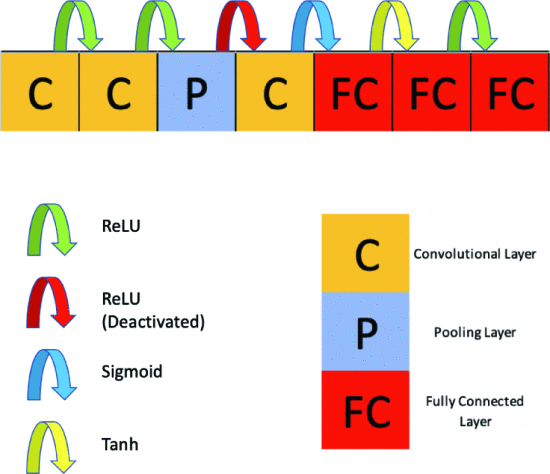Figure 4. example of CNN with the proposed gene encoding

Top 40% of individuals selected for tournament pool

Within this group

• Parents are randomly selected 20 times
• Crossover to generate next-generation (40% of population size)

20% of the population from within the pool by mutation using the best 40% of the previous generation and 40% of the children generated.

40 % : Best 40% of previous generation (to Tournament Pool)
40 % : Next generation generated by crossover
20 % : Next generation generated by Mutation

It is done until the maximum number of generations is reached, the optimal solution is selected, and full learning is done with at least 150 epochs (ne), a small batch size (bs), and a learning rate (lr) of 0.01 to 0.001. These flows are as in Algorithm 1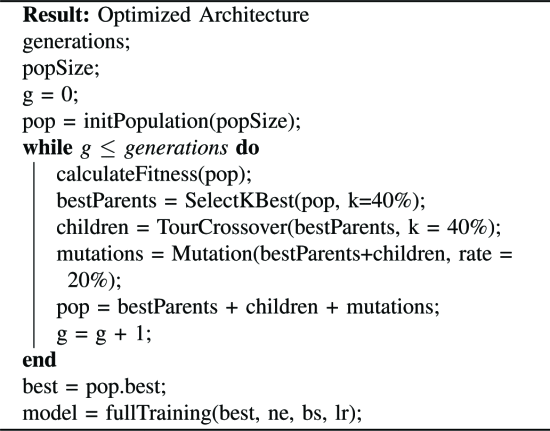Algorithm 1: Genetic Algorithm CNN

### D. Population Initialization

Population initialization

💡 F irst layer: convolutional layer with random parameters

No subsequent layer is specified, but it is always an all-bonded layer after the all-bonded layer The

*The number of all coupling layers is limited to 3 (from II-B)

The details of the initialization algorithm are as follows. The number of layers is taken randomly as minLayers, maxLayers, where the minimum and maximum of layers are defined.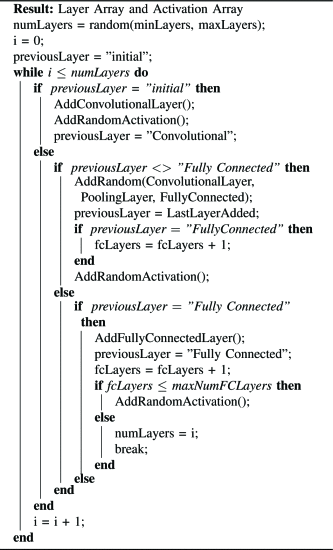### E. Crossover

The crossover proposed in this paper is as follows.

Apply tournament selections to parent individuals. The following is a list of the most common problems that can occur.

... and randomly select two pairs from the 40% best architecture candidates of the previous generation.

Figures 5 and 6 Crossover Operation

Generate two new architectural children by exchanging the same layer type between the two parents (the generated children are shown in Figure 6)

• C (the convolution layer) exchanges with C
• P (Pooling layer) exchanges with P
• FC (full coupling layer) exchanges with FC

If the parent layers don't match, no change (activity stays the same) The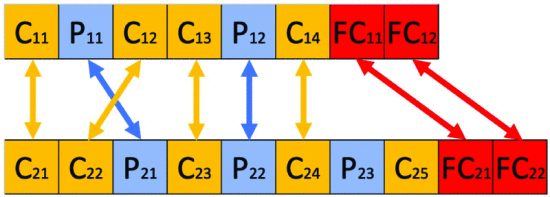Fig. 5 Crossover operation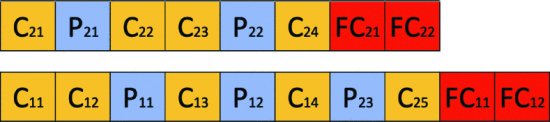Figure 6. child is generated using the example in Figure 5.

### F. Mutation

The following four types of mutations are defined

• Remove: "Remove" a layer at a random position
• Change: Randomly change a parameter of a layer ("Parameter change")
• Add Block: "Add Block" of "Convolutional Layer, Pooling Layer, ReLU" to the network architecture

The architecture to be mutated is randomly selected from the 40% best architecture and the 40% descendants, for a total mutation rate of 20%, generating the next generation

### G. Fitness

The Fitness function is computationally expensive because it requires the CNN to be trained The following example shows how to use the Therefore, only one epoch is used to compute fitness (so that GA can evaluate these networks within a day). When an optimal architecture is found at the end of the GA, it is fully trained to find the final Fitness of the optimized architecture The

## IV. Dataset

Selected two widely used benchmarks, MNIST and Fashion-MNIST

all

• 28x28 grayscale image
• 60,000 training images + 10,000 test images
• 10 classes

## V. Experimental Design

To evaluate the proposed algorithm, we compare it with other approaches.

Fitness functions are as follows The

$$fitness=\frac{f}{n}∗100,where \cdots(1)$$

• f: Number of correctly classified samples

• n: Number of samples

### Parameter Setting

The parameter settings are shown in the table below The following is a list of the available options.

• Fashion MNIST: Run GA 30 times

• MNIST: GA once

After running GA, the best architecture is FT (Fully Trained) (discussed in Section VI)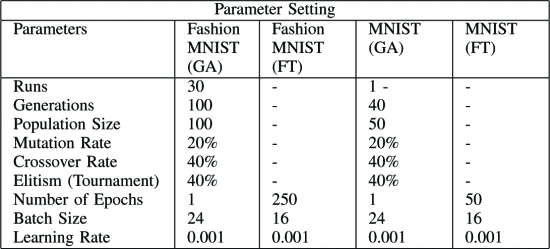The hybrid classification architecture is implemented with Python v3.6, PyTorch v1.4 framework and CUDA 10 accelerated with NVIDIA Tesla K80 GPU. Experiments were run on p3.2xlarge Amazon P3 instances

Model metrics

Evaluate "accuracy of all 10 classes" and "accuracy of each class" separately

## VI. results + discussion

### A) Fashion MNIST

The computational cost of the proposed method

• Each execution: about 18 hours
• Total experiment: 540 hours (GPU)

The value of the Fitness function is increasing with each generation. Reason. This is because the genetic operators used are well balanced between the search and use of the population in the pool of GAs The

What can be considered from Figure 7

• Accuracy skyrocketed in the first ten generations.
• Since then, there has been a slight increase.

Due to the constraint of the maximum number of layers that limits the search space

Wouldn't it be more valuable to increase the maximum number of layers in the initialization of the architecture than to increase the number of generations or populations?

Reason) Because the properties of Crossover and Mutation can be exploited to increase the diversity of the initial architecture and to better explore the search space.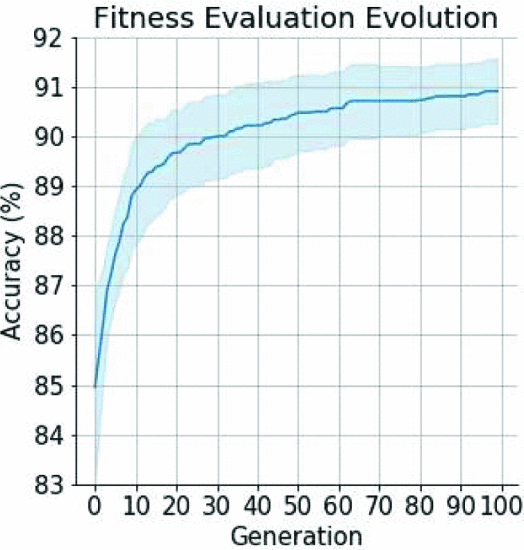Figure 7 Confidence intervals of the proposed algorithm on the Fashion MNIST dataset

Figure 8. What can be considered from

The results of Fully Trained on the Best 30 architectures found in the experiment are shown in Figure 8. The

Best network: 93.76% Worst network: 92.27

Mean accuracy of 93.13% with a 95% confidence interval (between 92.97% and 93.30%) → shows that GA-based methods can achieve stable accuracy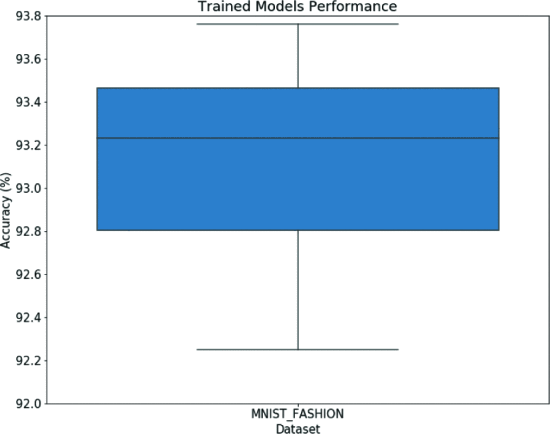Figure 8. optimal network architecture results after sufficient training (no outliers)

Table II. Optimal architecture for each class

• 96%+ accuracy for trousers, Sandal, sneakers, Bags, and Ankle Boot
• Low accuracy for Pullover, T-Shirt/top, Coat, and Shirt → As expected, accuracy is low because the images are similar.
• None of them had extremely low discrimination accuracy, and all of them were 79% or higher.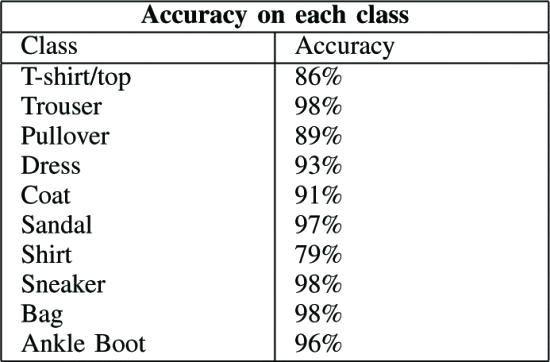Table II Accuracy by class

Comparison with other methods

The classification accuracy of the proposed method is denoted by (+) and that of the proposed method is denoted by (-) The

We were able to outperform the accuracy of 9 out of 13 methods

Compare with evoCNN

• the best accuracy is higher for evoCNN Is one of the factors that it uses a higher maximum number of layers than gaCNN?
• Mean accuracy is higher with gaCNN
→ gaCNN is more stable and can produce higher scores in a shorter time

The gaCNN runtime is about 18 hours (within a day ) using a GPU.

It is also useful in terms of time, considering that other architecture optimization methods take days or thousands of hours.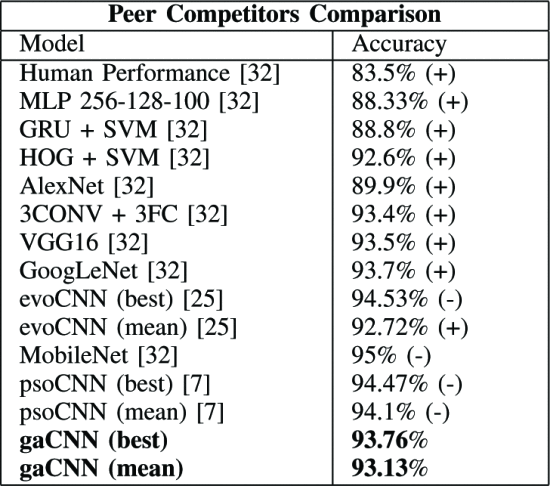Table III. the proposed algorithm performs better than most competitors on the test set

### B) MNIST

From the results of Fashion MNIST, we can say that gaCNN can produce stable results without outliers So, we run gaCNN only once to verify its accuracy.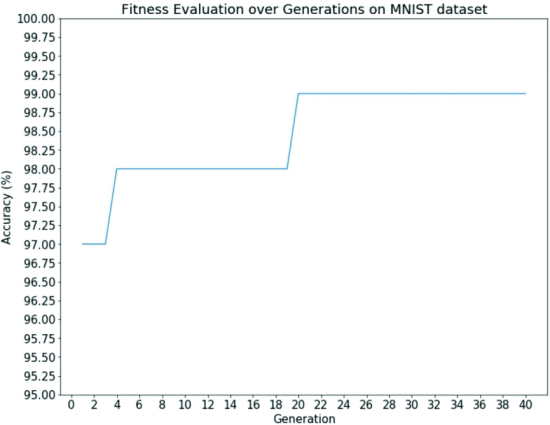Fig. 9. Results for the optimal network architecture after sufficient training

Table IV.

the class system, but without significant bias.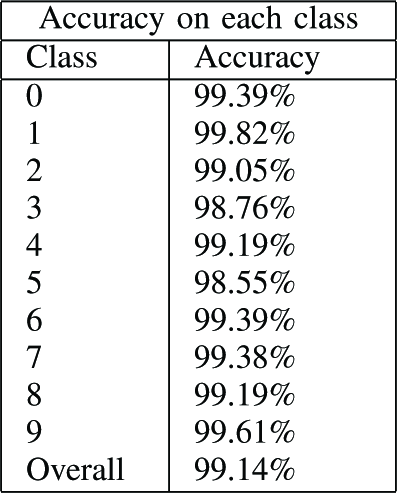TABLE IV Network Performance by class (Performance of optimized architecture after all training)

Table V: Comparison with other methods

The accuracy of gaCNN outperforms 12 out of 16 methods The

However, more runs are needed to provide confidence intervals for the proposed algorithm for this dataset.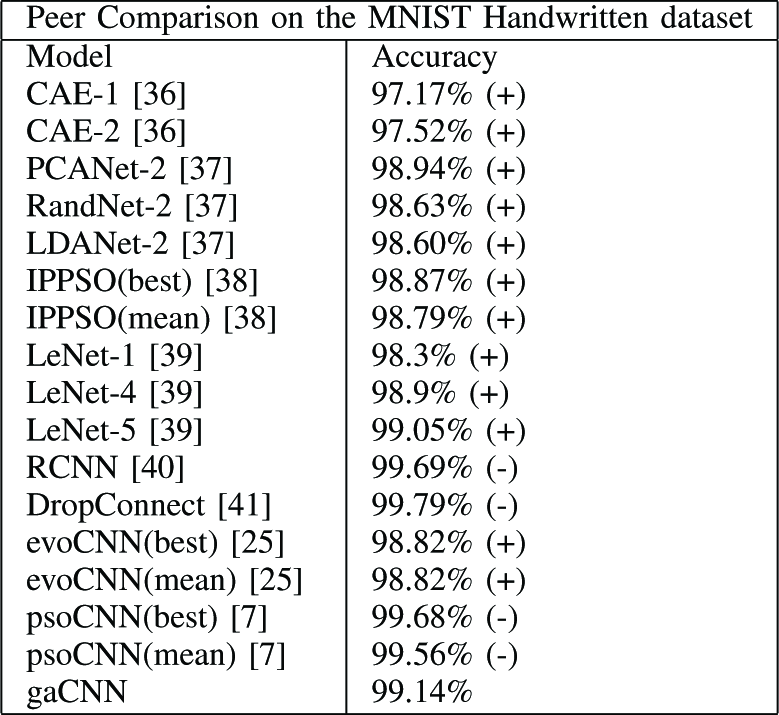TABLE V gaCNN performs better on the MNIST Handwritten dataset, outperforming 12 out of 16 competing methods in a single run (higher classification accuracy of the proposed method is denoted as (+), lower accuracy as (-))

## VII. conclusion & future works

We proposed a hybrid classification architecture consisting of CNNs optimized using GA. It utilizes a novel mutation operation and a network-wide heterogeneous activation function The

Algorithm: We were able to identify a competitive architecture using a less complex paradigm of connection and layer types. The number of days it took to identify the optimal NN was Only 1 day The

### Future Works

• More computing power
Can experiment further with more complex data sets such as CIFAR-10 and CIFAR-100
• Developed a surrogate model to approximate the fitness function
Could higher efficiency be achieved in the exploration of Deep Learning architectures?
• Application to other NN methods
Extendable to other network architectures, including different types of networks, as well as RNNs and RCNNs for other data sets

If you have any suggestions for improvement of the content of the article,